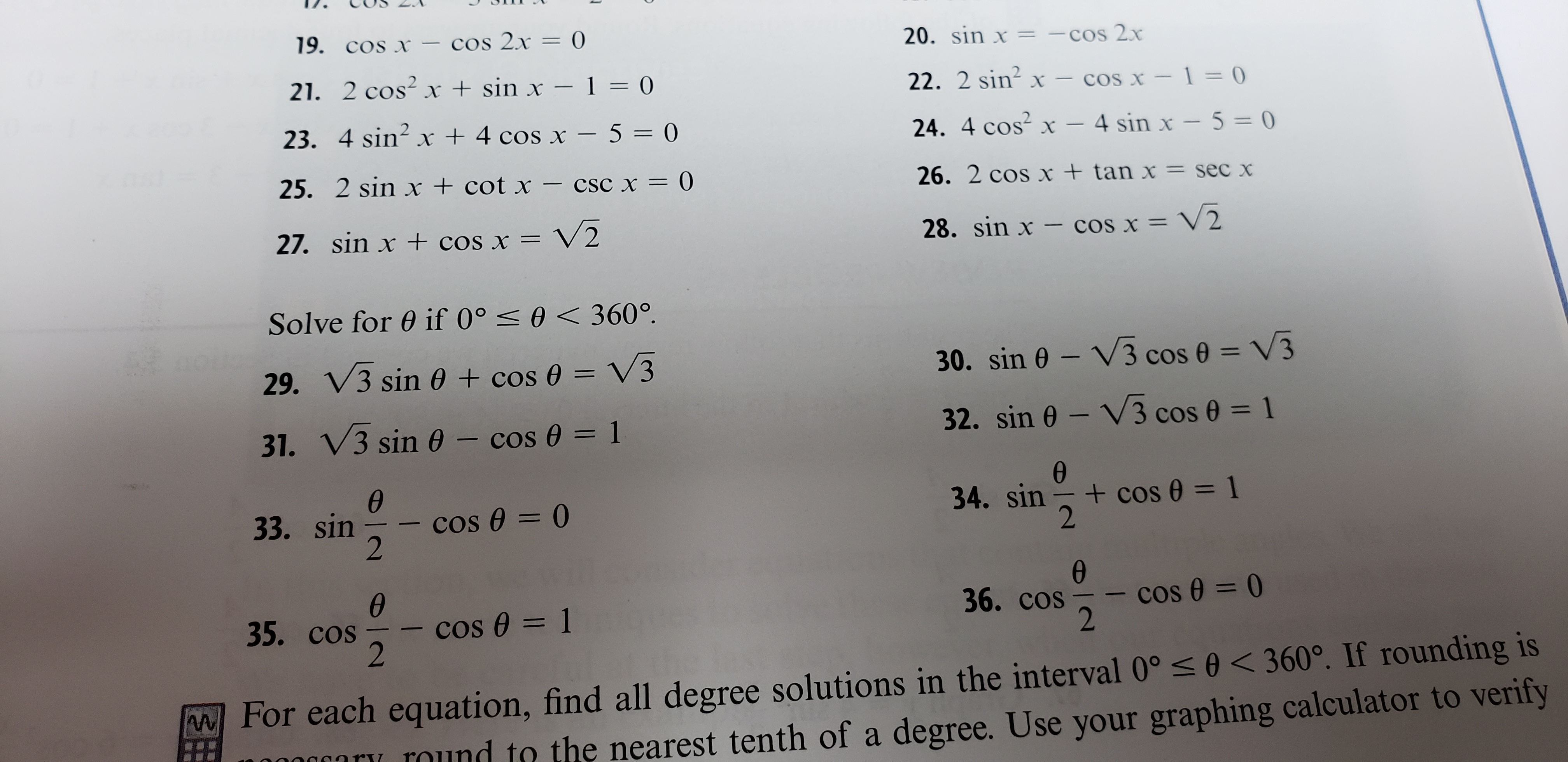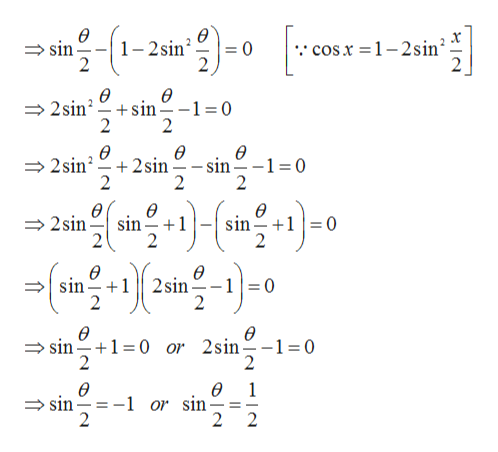# 19. cos x-cos 2x = 020. sin x -cos 2x221. 2 cos- x + sin x - 1 = 022. 2 sin2 xcos x 1=O23. 4 sin2 x +4 cos x - 5024. 4 cos x - 4 sin x - 5 = 025. 2 sin x + cot x- cSC x = )26. 2 cos x + tan x = sec xV227. sin x + cos x =28. sin x - cos x = V2Solve for 0 if 0°0 < 360°.V330. sin 0 V3 cos 029. V3 sin 0 + cos e = V332. sin 0 V3 cos 01V3 sin 0 -cos 0 131.34. sin cos = 12cos 0 = 0233. sincos e = 0236. coscos e 1235. cosFor each equation, find all degree solutions in the interval 0° 0

Question

Number 33help_outlineImage Transcriptionclose19. cos x-cos 2x = 0 20. sin x -cos 2x 2 21. 2 cos- x + sin x - 1 = 0 22. 2 sin2 x cos x 1=O 23. 4 sin2 x +4 cos x - 5 0 24. 4 cos x - 4 sin x - 5 = 0 25. 2 sin x + cot x- cSC x = ) 26. 2 cos x + tan x = sec x V2 27. sin x + cos x = 28. sin x - cos x = V2 Solve for 0 if 0° 0 < 360°. V3 30. sin 0 V3 cos 0 29. V3 sin 0 + cos e = V3 32. sin 0 V3 cos 0 1 V3 sin 0 -cos 0 1 31. 34. sin cos = 1 2 cos 0 = 0 2 33. sin cos e = 0 2 36. cos cos e 1 2 35. cos For each equation, find all degree solutions in the interval 0° 0<360°. If rounding is 0ary mund to the nearest tenth of a degree. Use your graphing calculator to verify # fullscreen
check_circleExpert Solution
Step 1

Given,

Step 2

On simplificatio...help_outlineImage Transcriptioncloseө 1-2sin 2 cosx = 1-2sin 2 sin 0 2 ө ө +sin 2 2sin =0 2 ө 2sin 2 sin 2 ө - sin 2 -1 0 2 ө ө sin +1 2 Ө 2sin sin +10 Ө ө 10 sin 2sin 1 2 2 ө sin 1 0 or 2sin 2 ө = 0 2 ө -1 or sin 2 ө 1 sin 2 2 fullscreen

### Want to see the full answer?

See Solution

#### Want to see this answer and more?

Solutions are written by subject experts who are available 24/7. Questions are typically answered within 1 hour*

See Solution
*Response times may vary by subject and question
Tagged in

### Trigonometric Ratios